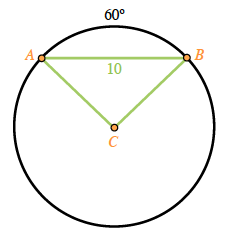### Home > CCG > Chapter 11 > Lesson 11.1.1 > Problem11-20

11-20.

In a circle, chord $\overline{AB}$ has length $10$ units, while $m\overarc{AB} = 60°$. What is the area of the circle? Draw a diagram and show all work.

The measure of the intercepted arc is equal to the measure of the central angle.
$m\overarc{AB} = 60° = m∠C$

$AC=BC$, because both are radii, so $ΔABC$ is isosceles.

Isosceles triangles have congruent base angles, so $m∠A = m∠B$.

$m∠A + m∠B + m∠C = 180°$
but $m∠C = 60°$, $m∠A = m∠B$
so $m∠B + m∠B + 60° = 180°$
$2m∠B = 120°$
$m∠B = 60° = m∠A$$π(10)^2 = 100π \approx 314.16\text{ units}^2$

Because the angles are all congruent, the triangle is equilateral.

So the length of the radius ($AC$) is $10$.